# RD Sharma Solutions for Class 10 Maths Chapter 15 Areas Related To Circles Exercise 15.4

In our everyday lives, we come across many plane figures which are combinations of two or more plane figures. This exercise contains problems on finding the area of such combinations of plane figures. The RD Sharma Solutions Class 10 prepared by experts at BYJU’S can be utilised by students to access detailed solutions to all exercise problems. Also, students can access the RD Sharma Solutions for Class 10 Maths Chapter 15 Areas Related To Circles Exercise 15.4 PDF provided below.

## RD Sharma Solutions for Class 10 Maths Chapter 15 Areas Related To Circles Exercise 15.4 Download PDF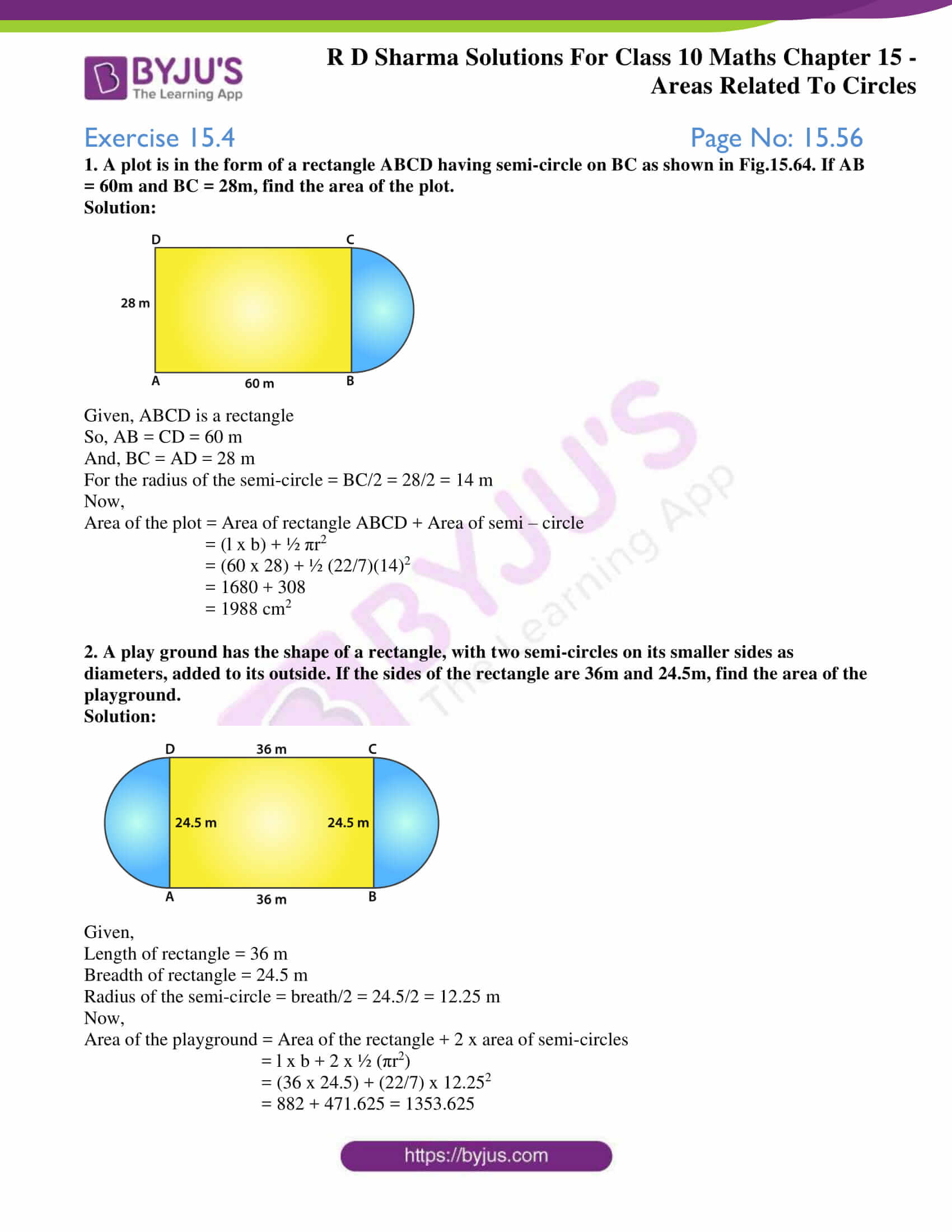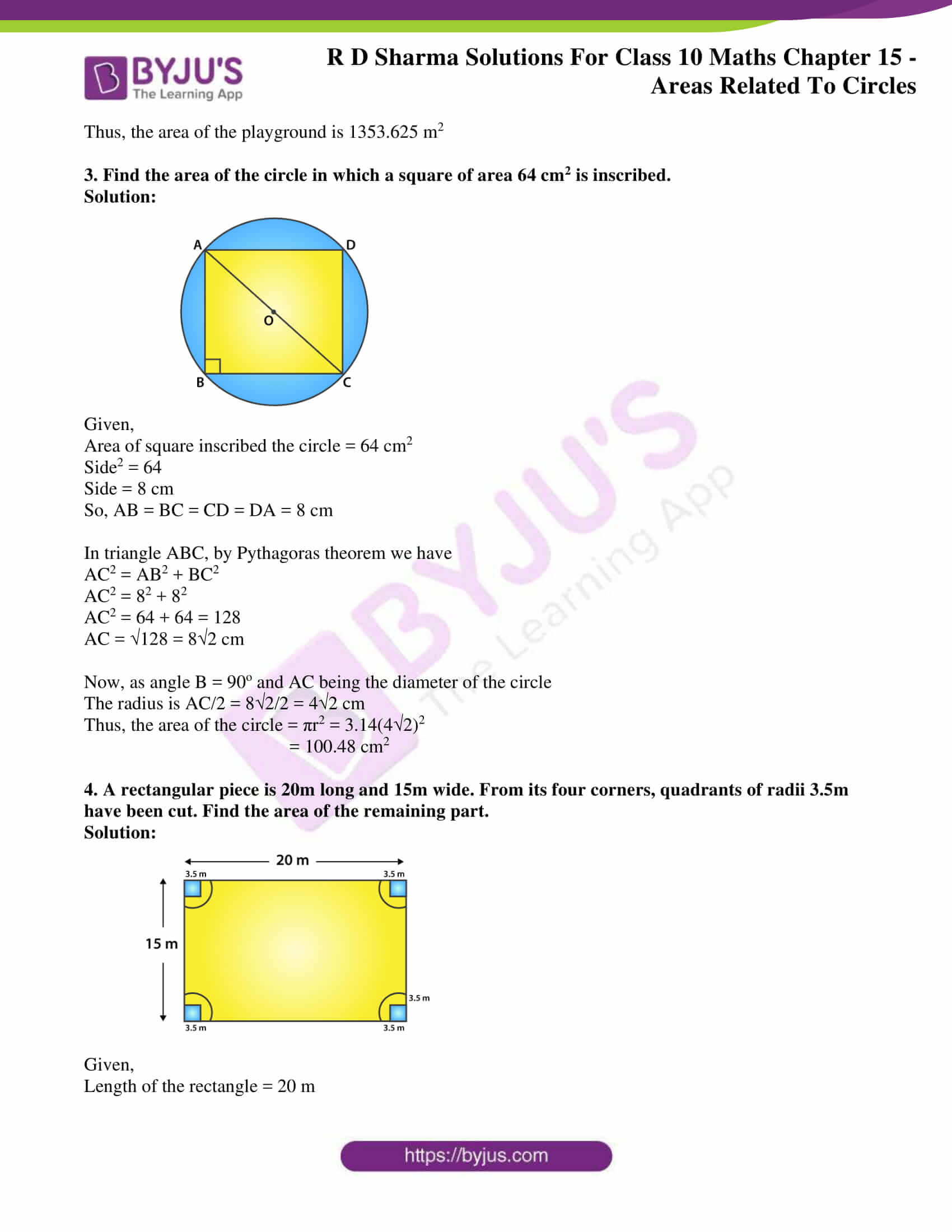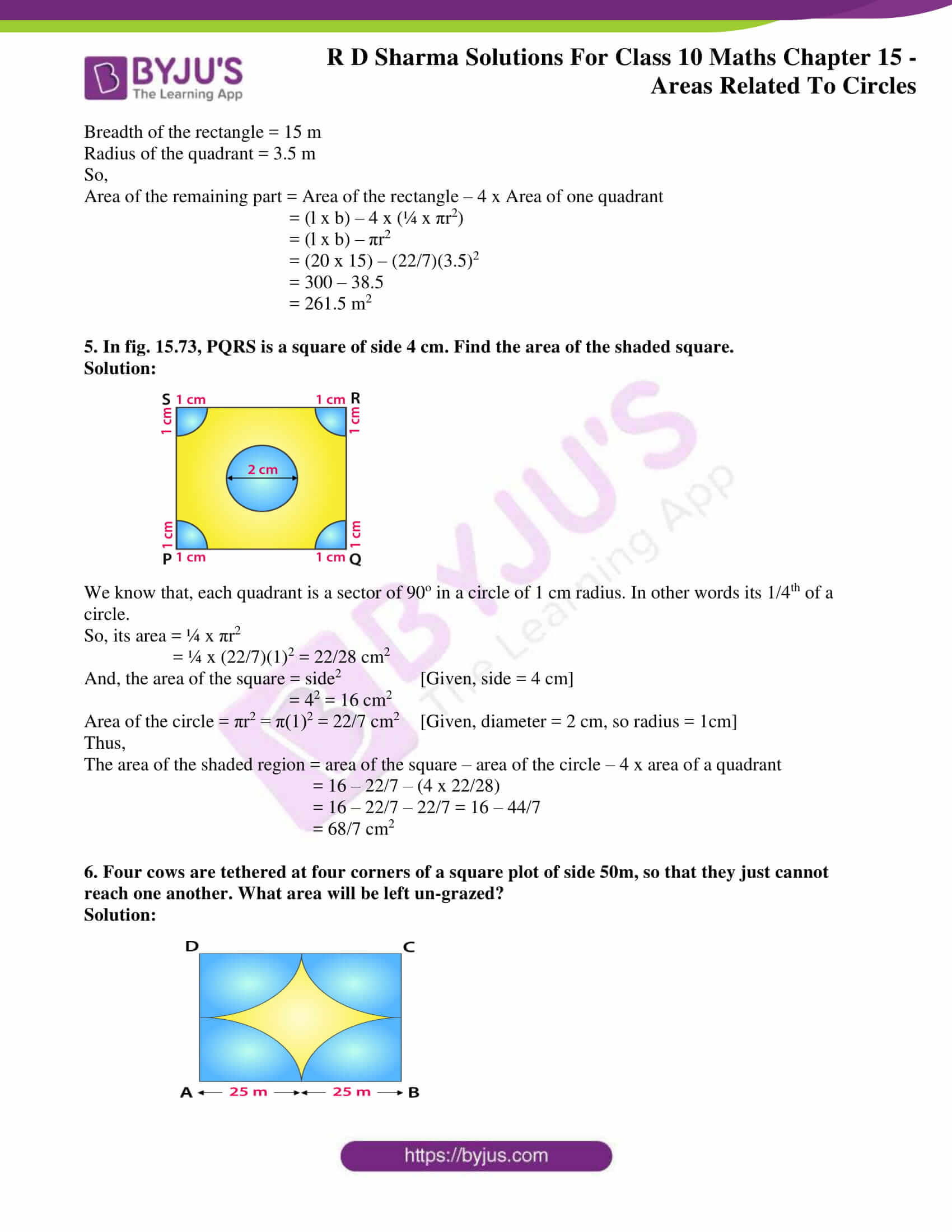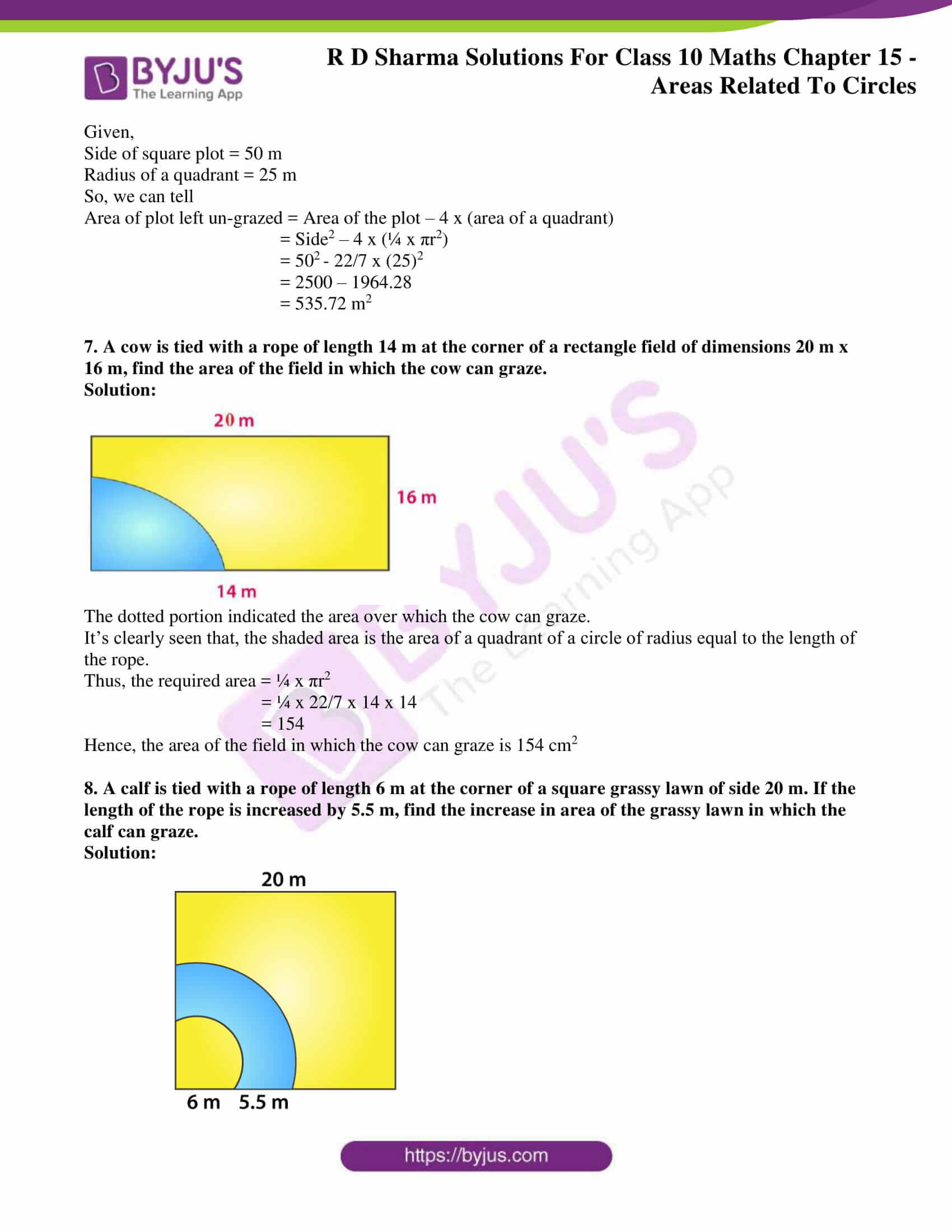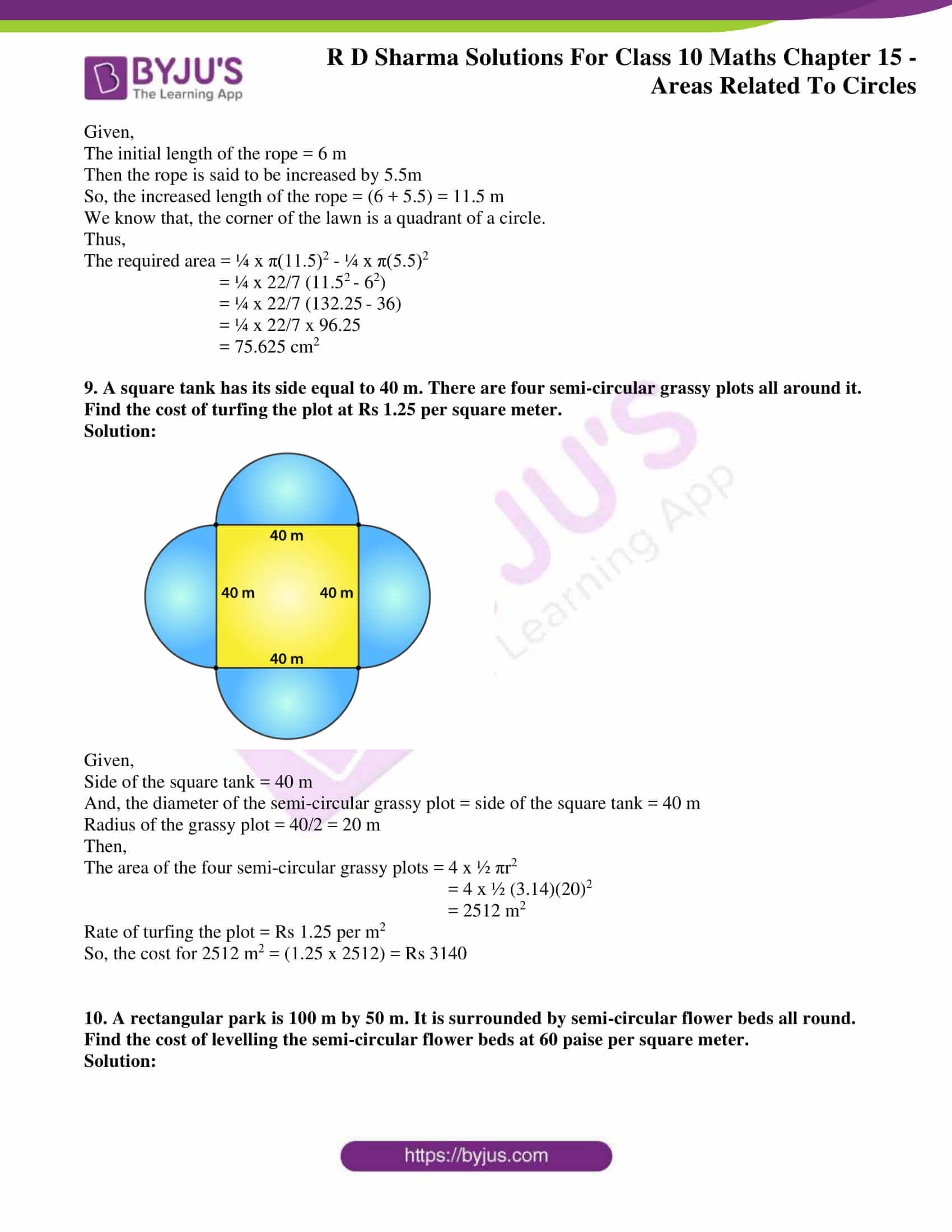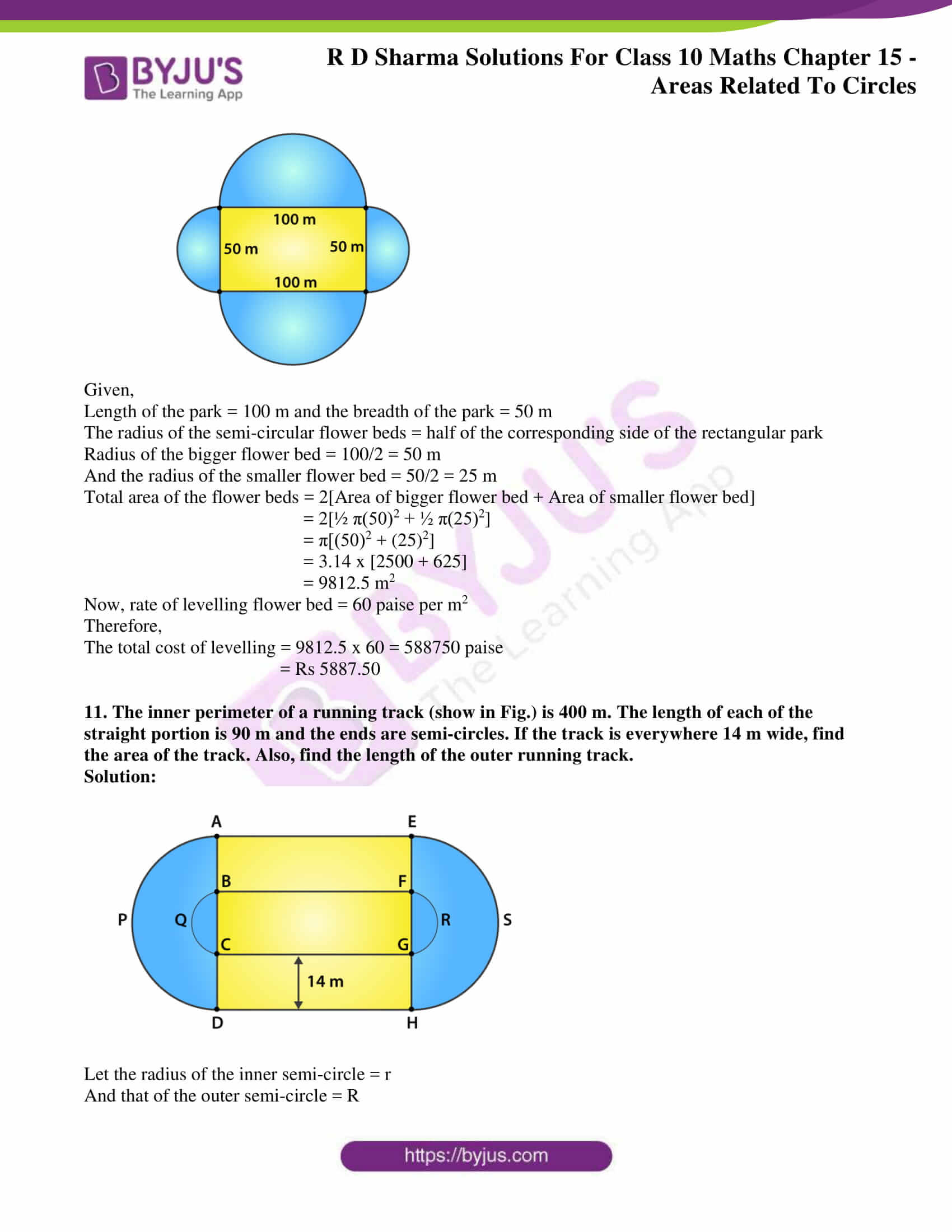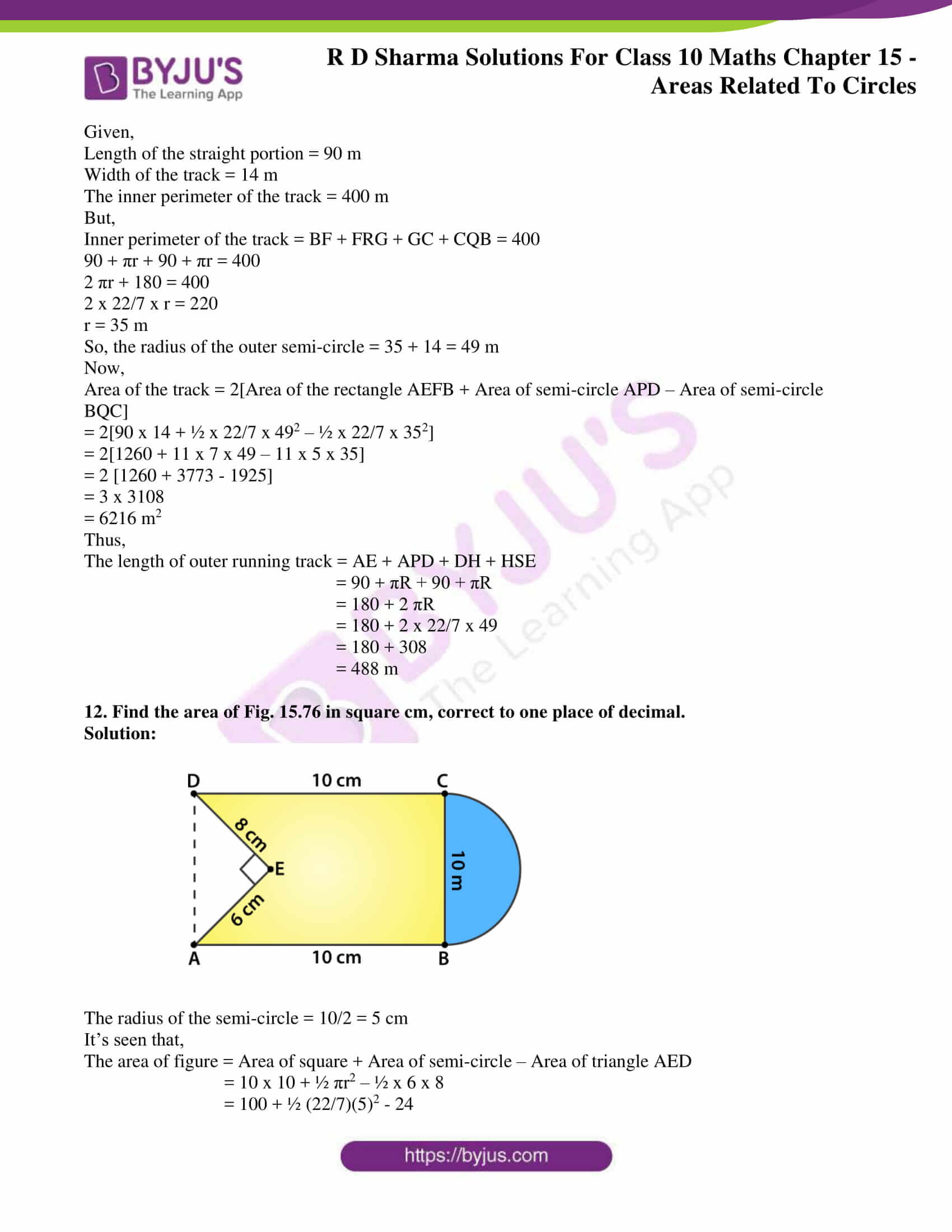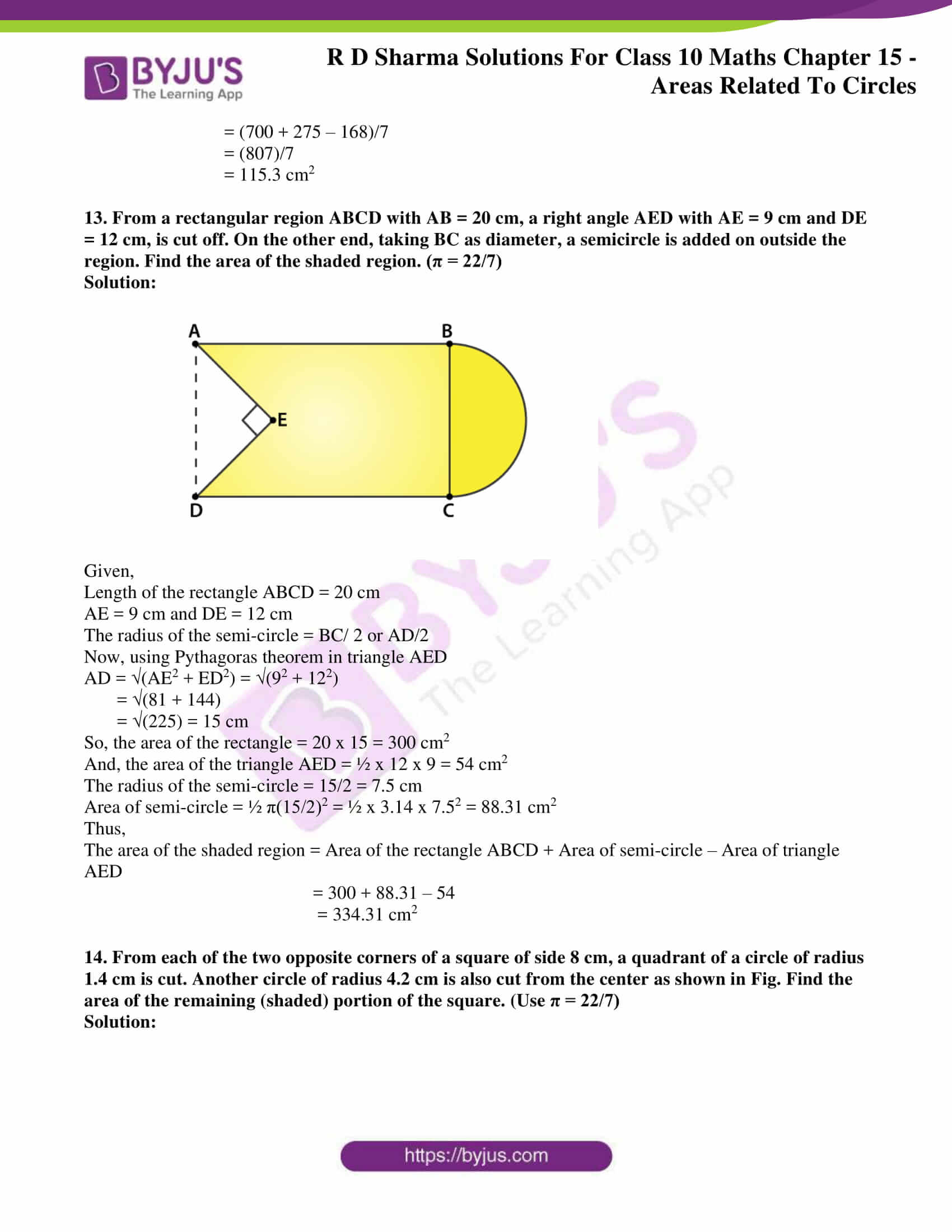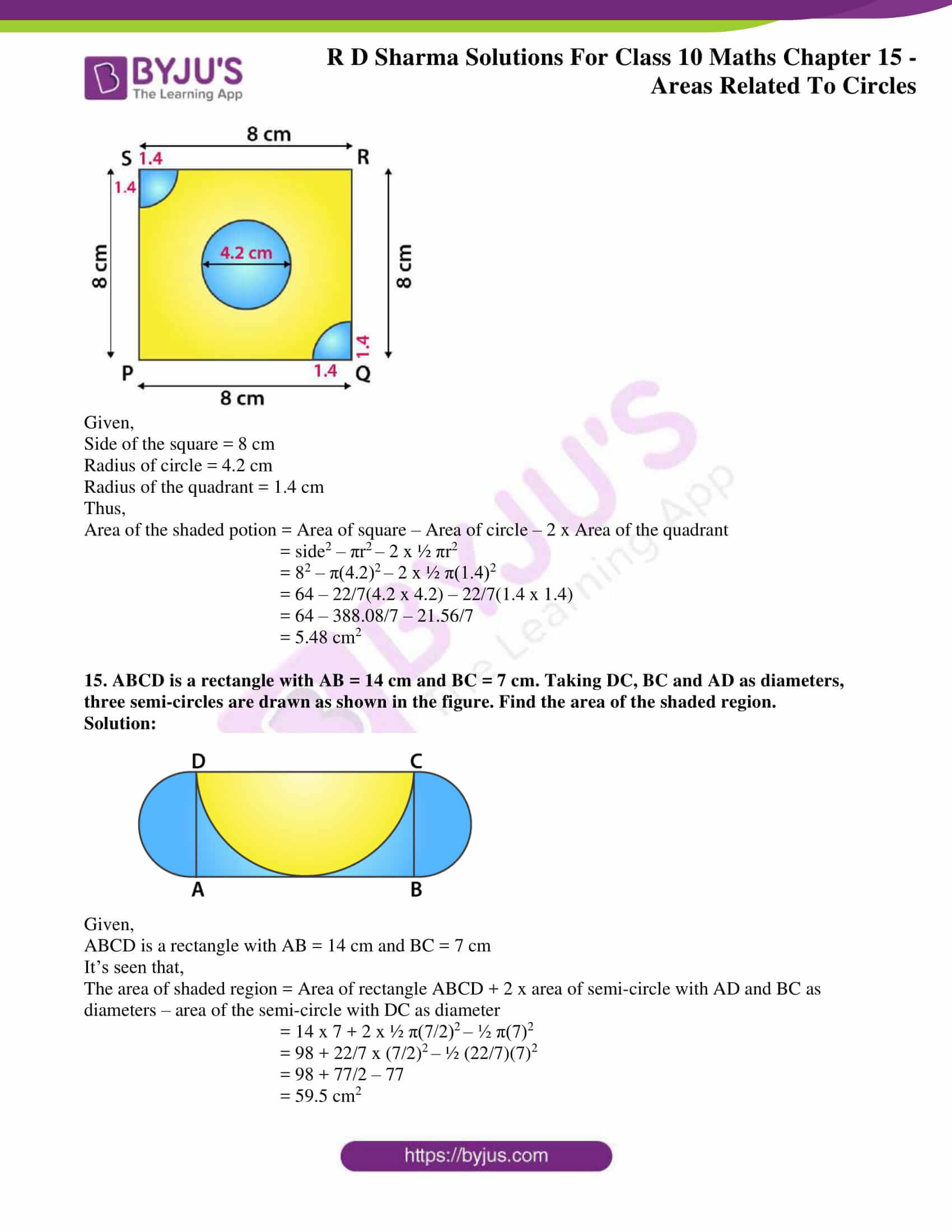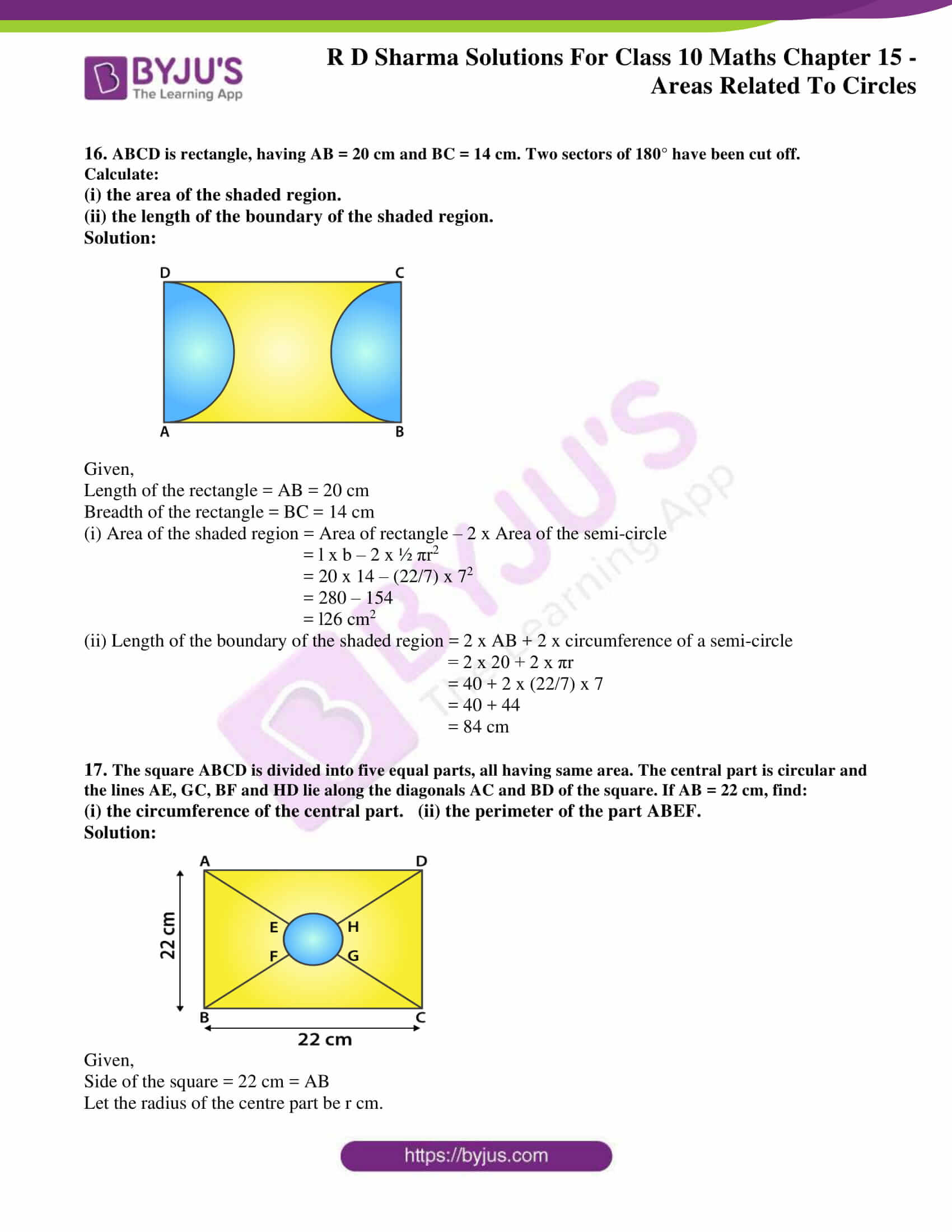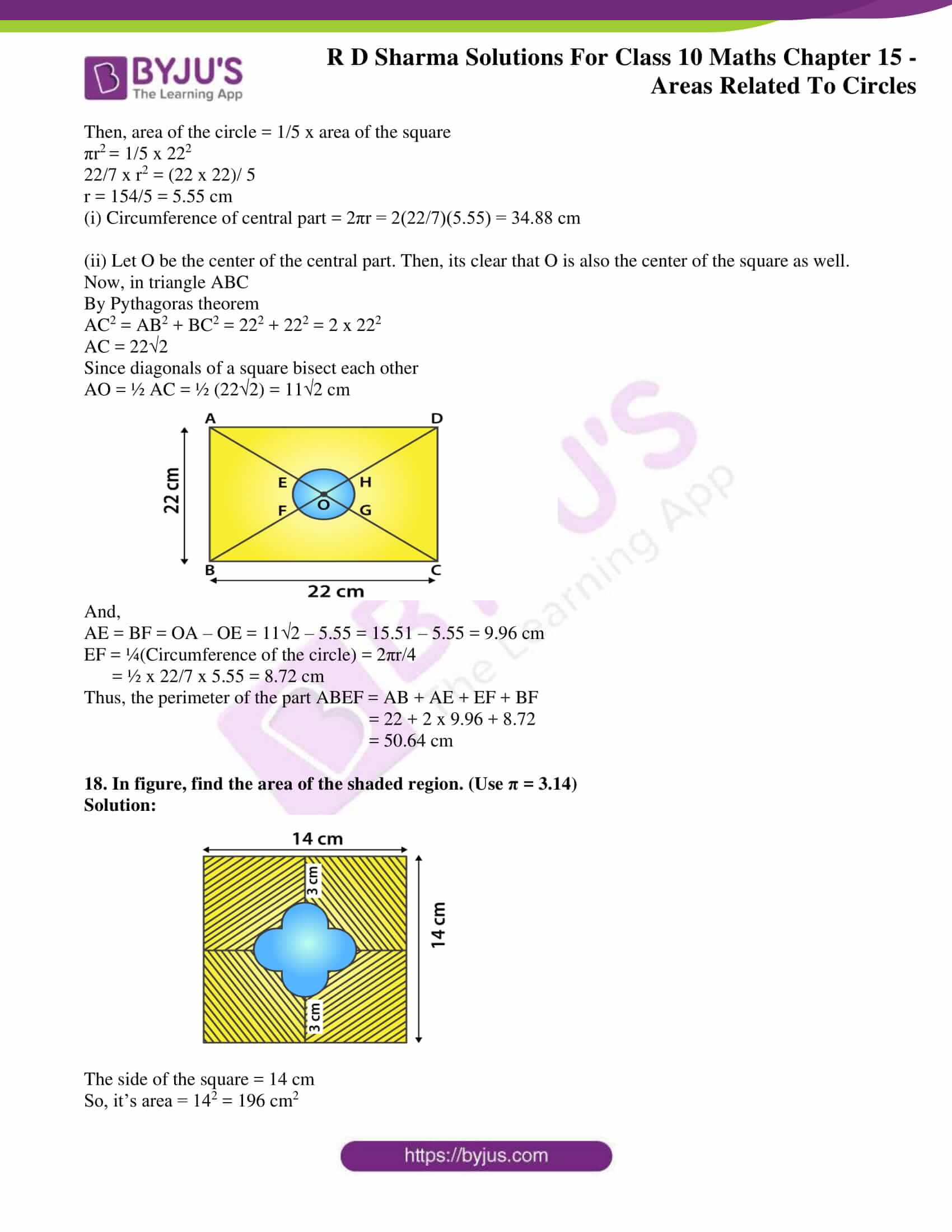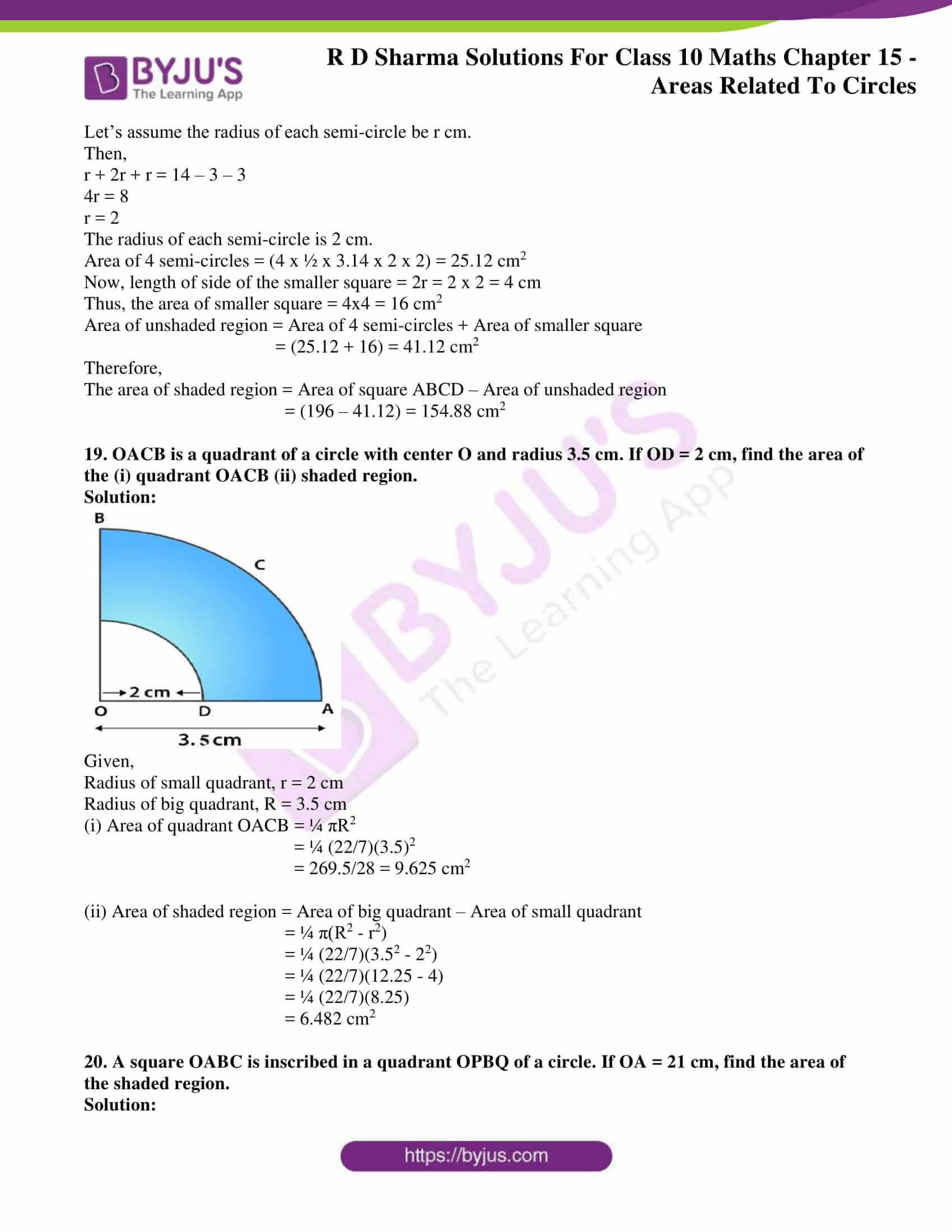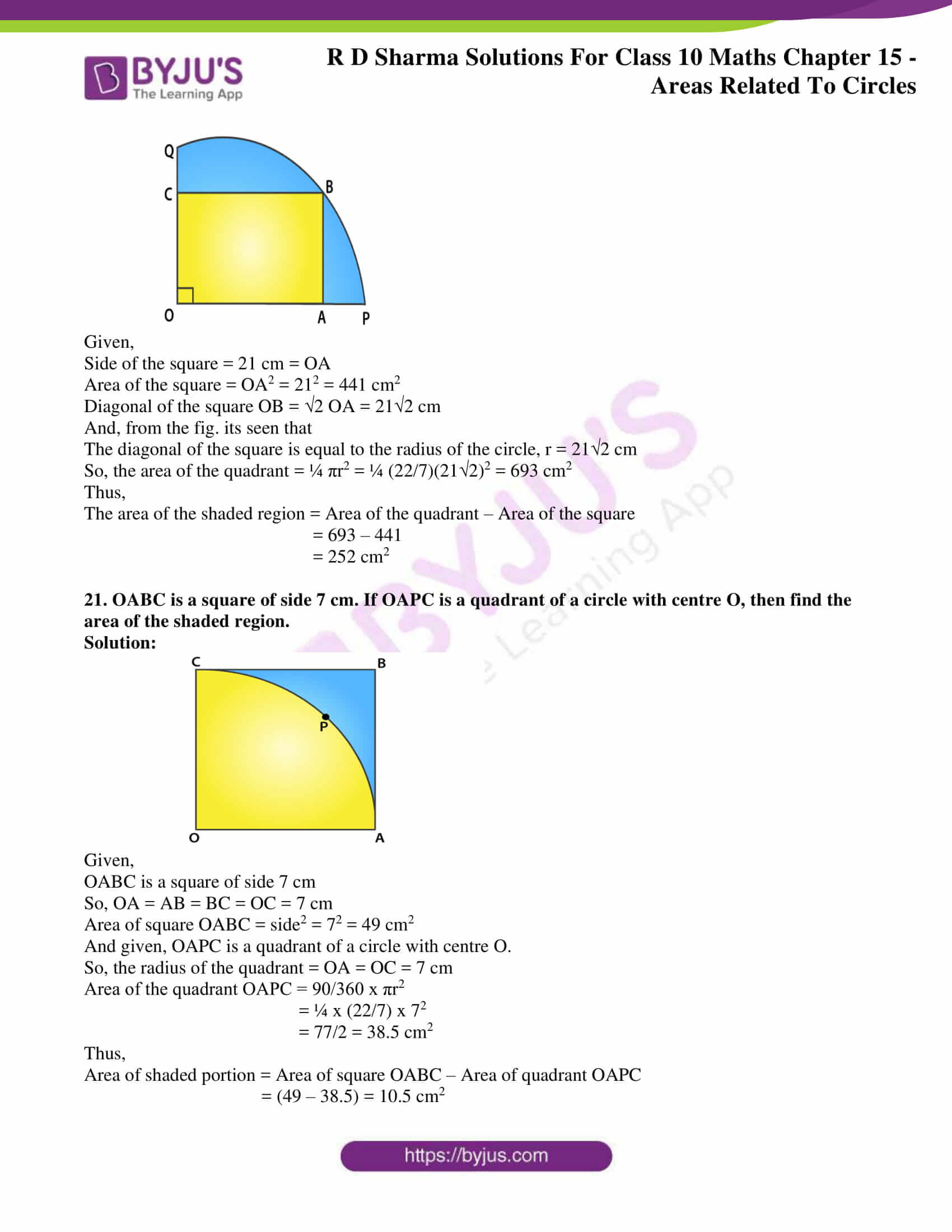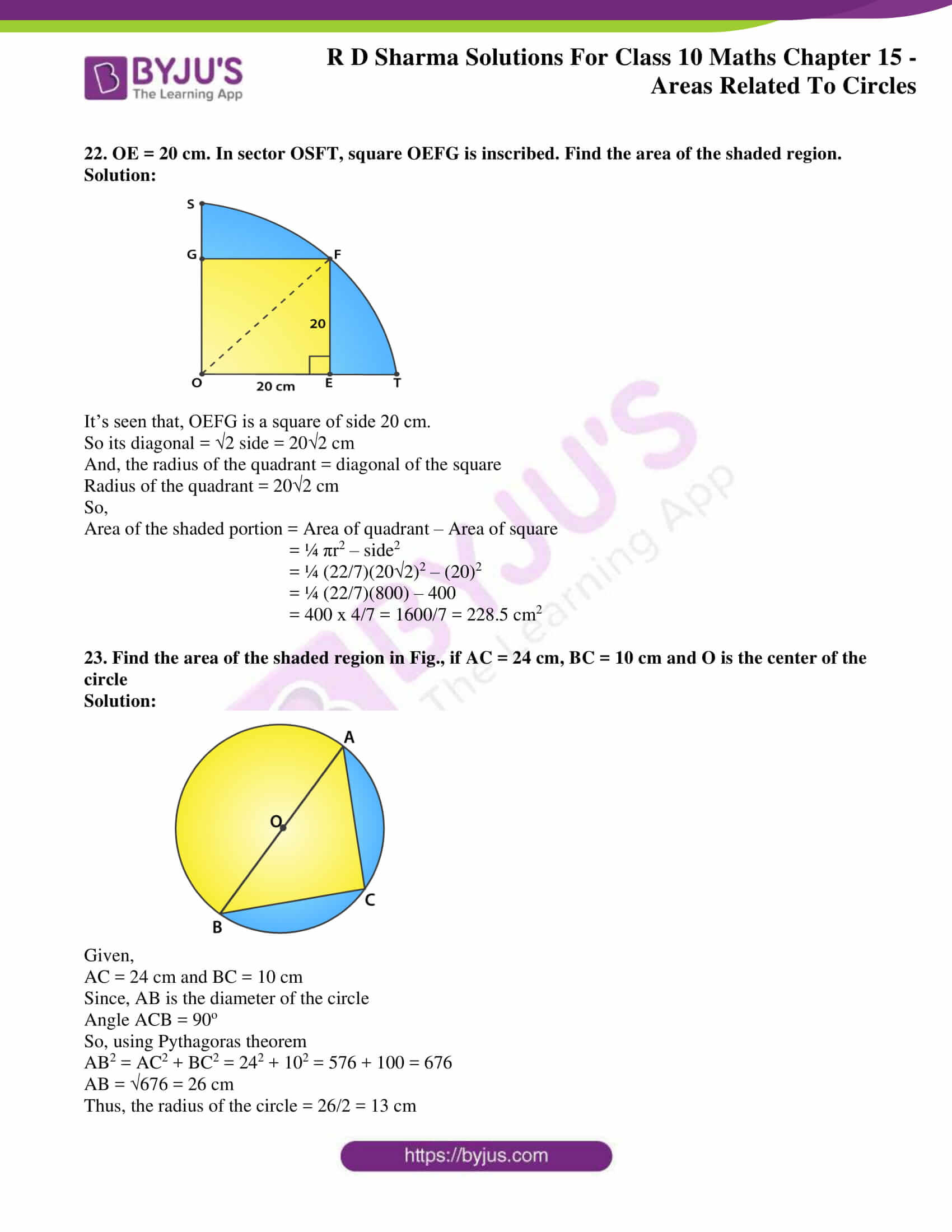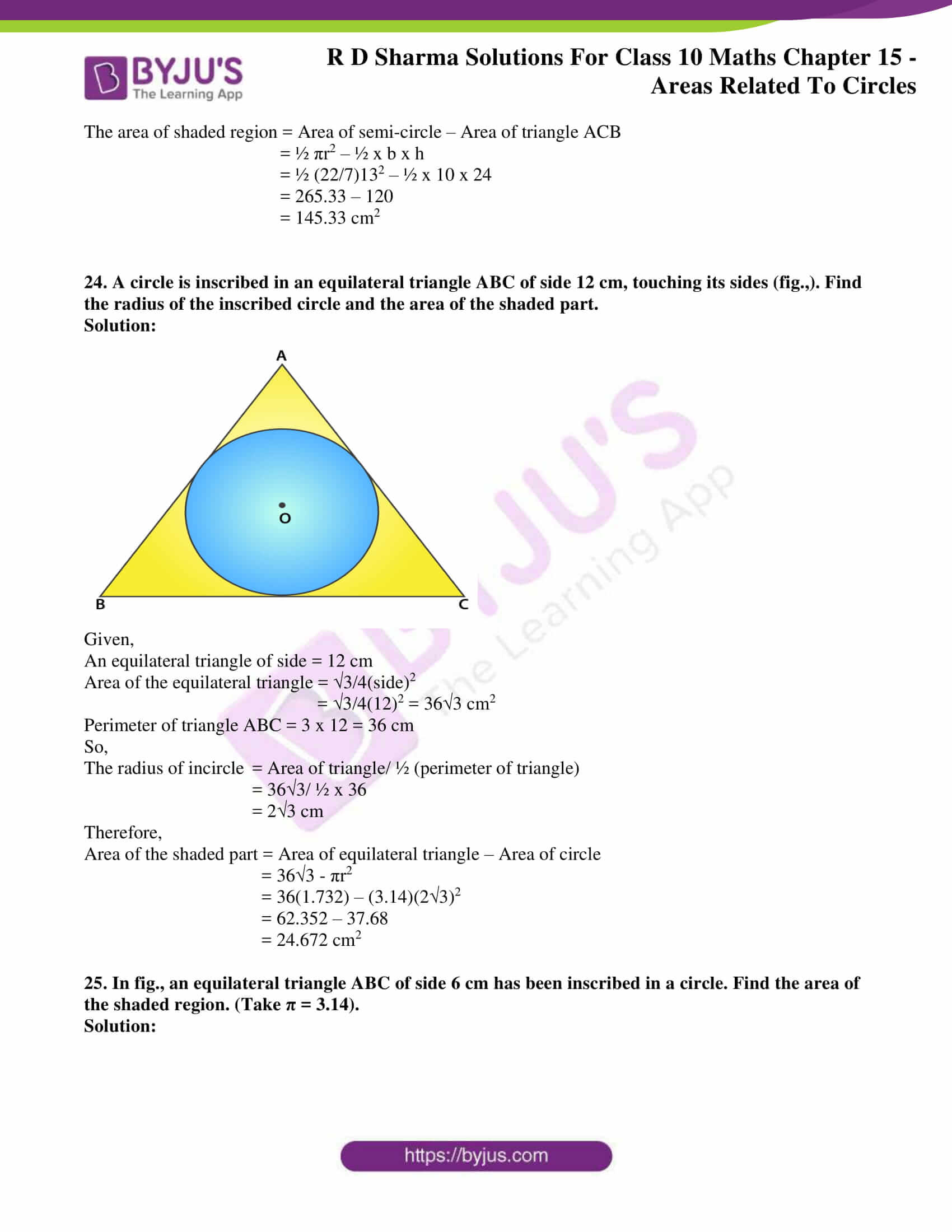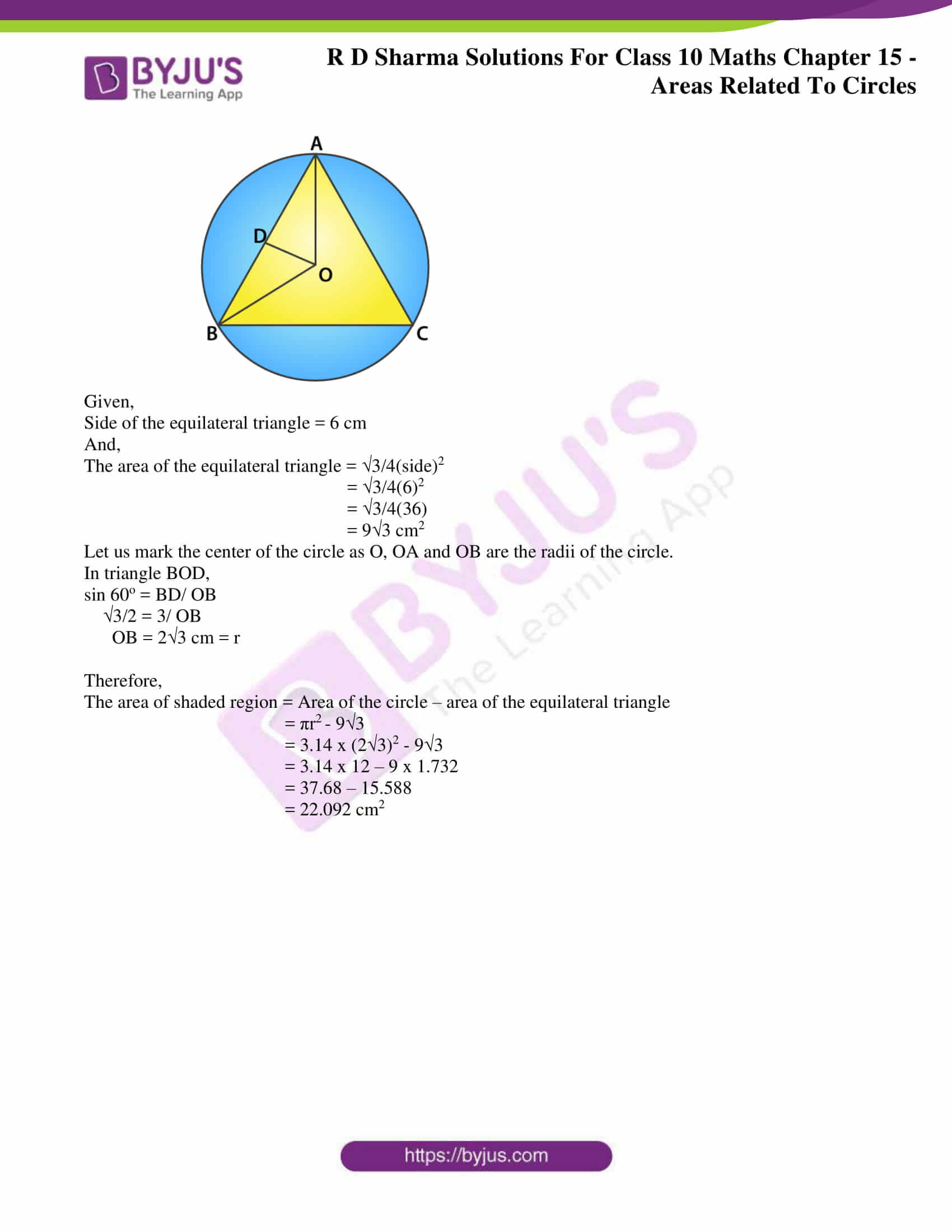### Access RD Sharma Solutions for Class 10 Maths Chapter 15 Areas Related To Circles Exercise 15.4

1. A plot is in the form of a rectangle ABCD having semi-circle on BC as shown in Fig.15.64. If AB = 60m and BC = 28m, find the area of the plot.

Solution: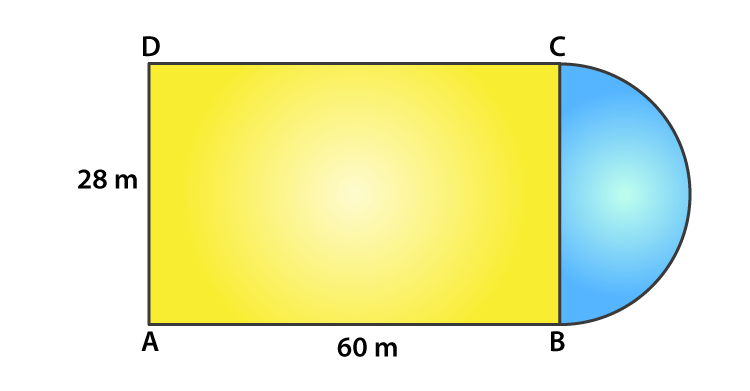Given, ABCD is a rectangle

So, AB = CD = 60 m

And, BC = AD = 28 m

For the radius of the semi-circle = BC/2 = 28/2 = 14 m

Now,

Area of the plot = Area of rectangle ABCD + Area of semi – circle

= (l x b) + ½ πr2

= (60 x 28) + ½ (22/7)(14)2

= 1680 + 308

= 1988 cm2

2. A play ground has the shape of a rectangle, with two semi-circles on its smaller sides as diameters, added to its outside. If the sides of the rectangle are 36m and 24.5m, find the area of the playground.

Solution: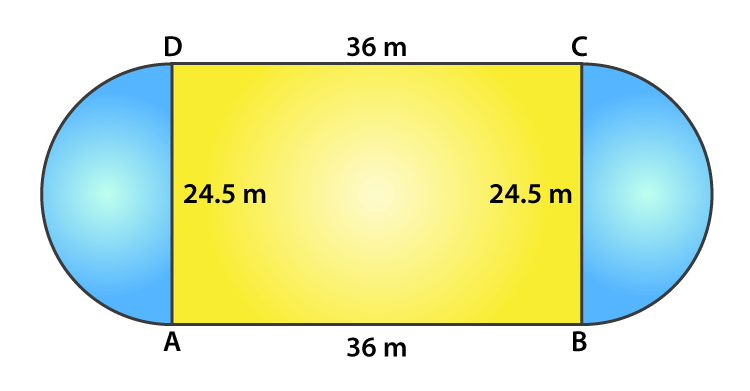Given,

Length of rectangle = 36 m

Breadth of rectangle = 24.5 m

Radius of the semi-circle = breath/2 = 24.5/2 = 12.25 m

Now,

Area of the playground = Area of the rectangle + 2 x area of semi-circles

= l x b + 2 x ½ (πr2)

= (36 x 24.5) + (22/7) x 12.252

= 882 + 471.625 = 1353.625

Thus, the area of the playground is 1353.625 m2

3. Find the area of the circle in which a square of area 64 cm2 is inscribed.

Solution: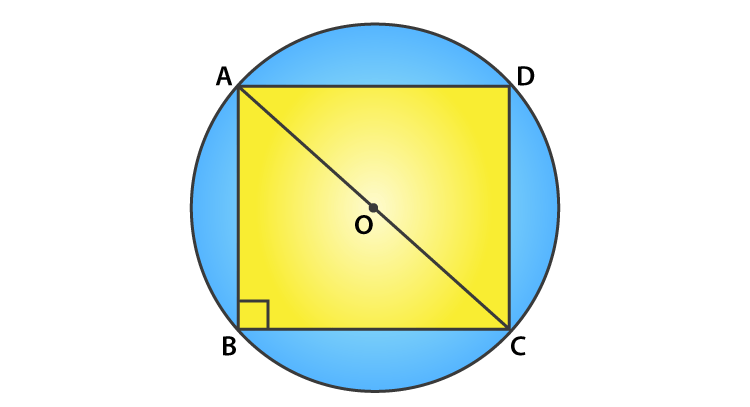Given,

Area of square inscribed the circle = 64 cm2

Side2 = 64

Side = 8 cm

So, AB = BC = CD = DA = 8 cm

In triangle ABC, by Pythagoras theorem we have

AC2 = AB2 + BC2

AC2 = 82 + 82

AC2 = 64 + 64 = 128

AC = √128 = 8√2 cm

Now, as angle B = 90o and AC being the diameter of the circle

The radius is AC/2 = 8√2/2 = 4√2 cm

Thus, the area of the circle = πr2 = 3.14(4√2)2

= 100.48 cm2

4. A rectangular piece is 20m long and 15m wide. From its four corners, quadrants of radii 3.5m have been cut. Find the area of the remaining part.

Solution: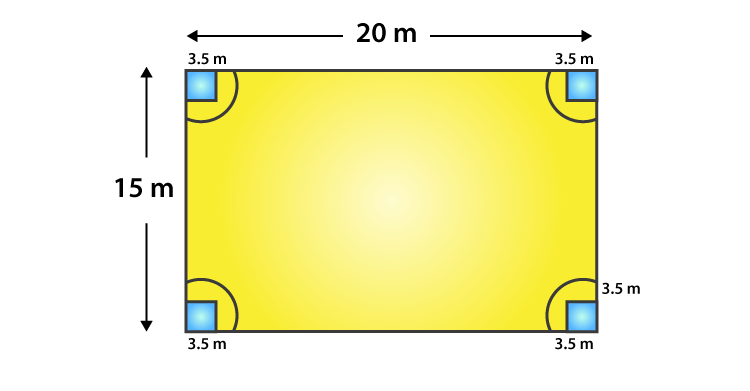Given,

Length of the rectangle = 20 m

Breadth of the rectangle = 15 m

So,

Area of the remaining part = Area of the rectangle – 4 x Area of one quadrant

= (l x b) – 4 x (¼ x πr2)

= (l x b) – πr2

= (20 x 15) – (22/7)(3.5)2

= 300 – 38.5

= 261.5 m2

5. In fig. 15.73, PQRS is a square of side 4 cm. Find the area of the shaded square.

Solution: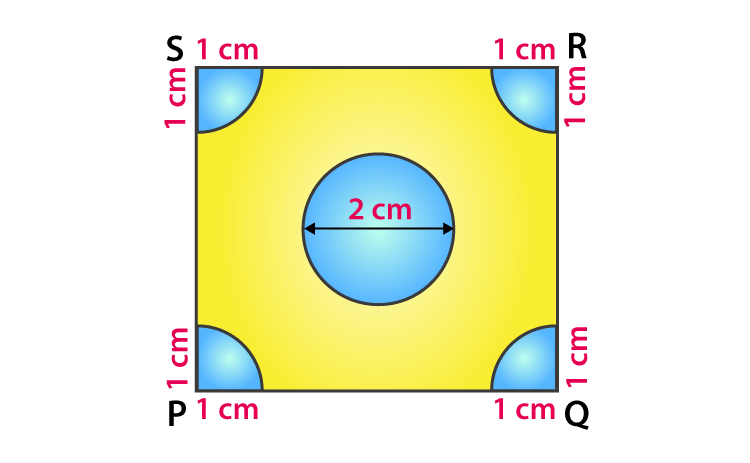We know that, each quadrant is a sector of 90o in a circle of 1 cm radius. In other words its 1/4th of a circle.

So, its area = ¼ x πr2

= ¼ x (22/7)(1)2 = 22/28 cm2

And, the area of the square = side2 [Given, side = 4 cm]

= 42 = 16 cm2

Area of the circle = πr2 = π(1)2 = 22/7 cm2  [Given, diameter = 2 cm, so radius = 1cm]

Thus,

The area of the shaded region = area of the square – area of the circle – 4 x area of a quadrant

= 16 – 22/7 – (4 x 22/28)

= 16 – 22/7 – 22/7 = 16 – 44/7

= 68/7 cm2

6. Four cows are tethered at four corners of a square plot of side 50m, so that they just cannot reach one another. What area will be left un-grazed?

Solution: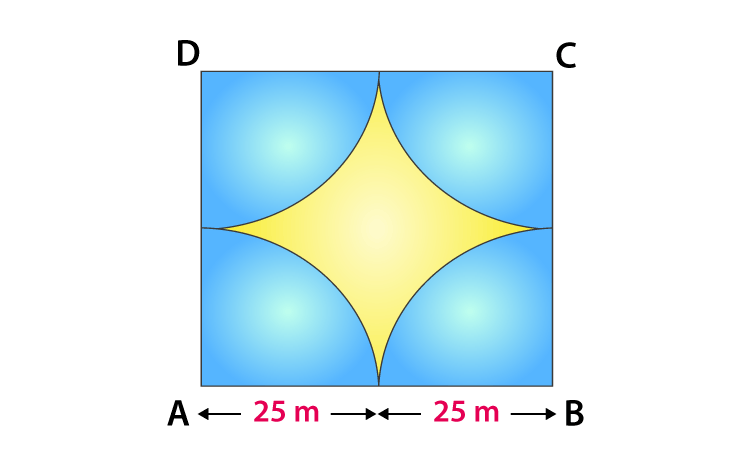Given,

Side of square plot = 50 m

So, we can tell

Area of plot left un-grazed = Area of the plot – 4 x (area of a quadrant)

= Side2 – 4 x (¼ x πr2)

= 502 – 22/7 x (25)2

= 2500 – 1964.28

= 535.72 m2

7. A cow is tied with a rope of length 14 m at the corner of a rectangle field of dimensions 20 m x 16 m, find the area of the field in which the cow can graze.

Solution: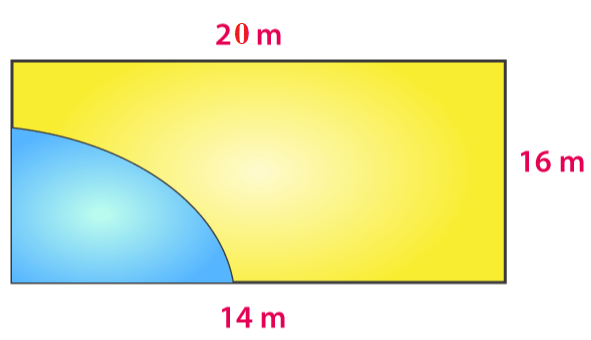The dotted portion indicated the area over which the cow can graze.

It’s clearly seen that, the shaded area is the area of a quadrant of a circle of radius equal to the length of the rope.

Thus, the required area = ¼ x πr2

= ¼ x 22/7 x 14 x 14

= 154

Hence, the area of the field in which the cow can graze is 154 cm2

8. A calf is tied with a rope of length 6 m at the corner of a square grassy lawn of side 20 m. If the length of the rope is increased by 5.5 m, find the increase in area of the grassy lawn in which the calf can graze.

Solution: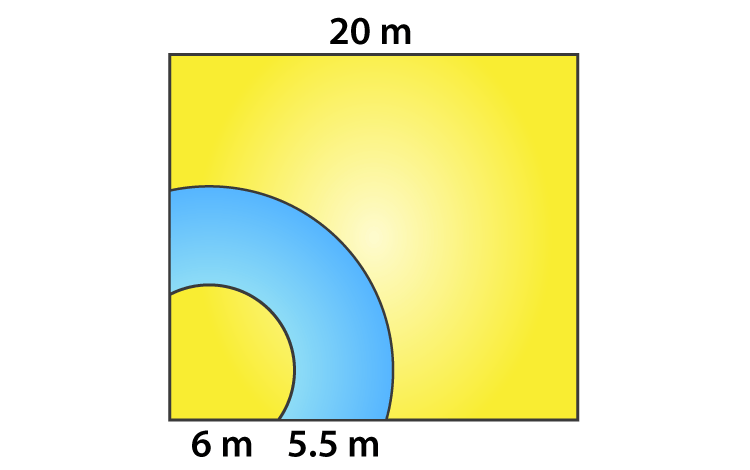Given,

The initial length of the rope = 6 m

Then the rope is said to be increased by 5.5m

So, the increased length of the rope = (6 + 5.5) = 11.5 m

We know that, the corner of the lawn is a quadrant of a circle.

Thus,

The required area = ¼ x π(11.5)2 – ¼ x π(5.5)2

= ¼ x 22/7 (11.52 – 62)

= ¼ x 22/7 (132.25 – 36)

= ¼ x 22/7 x 96.25

= 75.625 cm2

9. A square tank has its side equal to 40 m. There are four semi-circular grassy plots all around it. Find the cost of turfing the plot at Rs 1.25 per square meter.

Solution: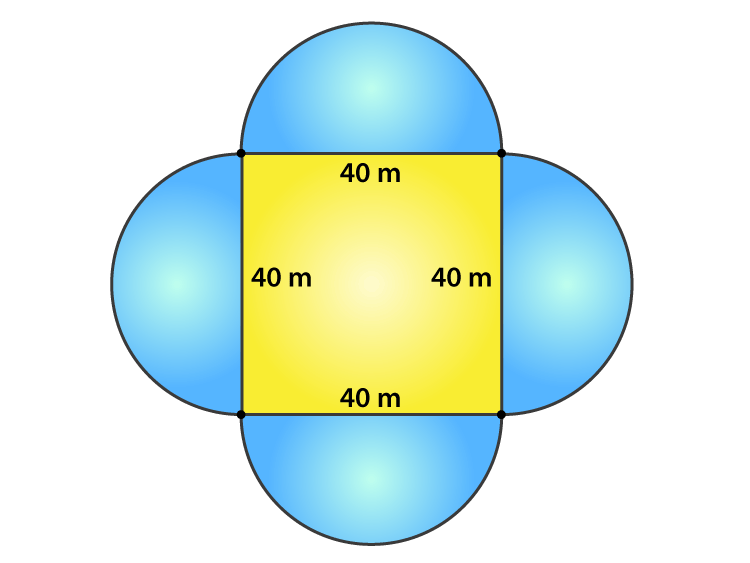Given,

Side of the square tank = 40 m

And, the diameter of the semi-circular grassy plot = side of the square tank = 40 m

Radius of the grassy plot = 40/2 = 20 m

Then,

The area of the four semi-circular grassy plots = 4 x ½ πr2

= 4 x ½ (3.14)(20)2

= 2512 m2

Rate of turfing the plot = Rs 1.25 per m2

So, the cost for 2512 m2 = (1.25 x 2512) = Rs 3140

10. A rectangular park is 100 m by 50 m. It is surrounded by semi-circular flower beds all round. Find the cost of levelling the semi-circular flower beds at 60 paise per square meter.

Solution: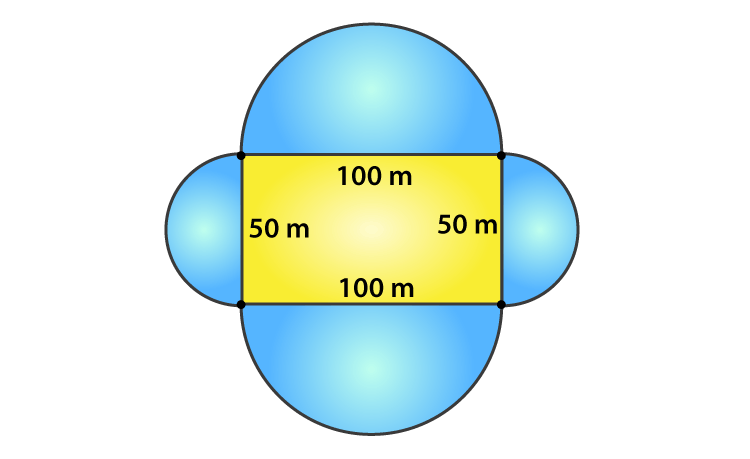Given,

Length of the park = 100 m and the breadth of the park = 50 m

The radius of the semi-circular flower beds = half of the corresponding side of the rectangular park

Radius of the bigger flower bed = 100/2 = 50 m

And the radius of the smaller flower bed = 50/2 = 25 m

Total area of the flower beds = 2[Area of bigger flower bed + Area of smaller flower bed]

= 2[½ π(50)2 + ½ π(25)2]

= π[(50)2 + (25)2]

= 3.14 x [2500 + 625]

= 9812.5 m2

Now, rate of levelling flower bed = 60 paise per m2

Therefore,

The total cost of levelling = 9812.5 x 60 = 588750 paise

= Rs 5887.50

11. The inner perimeter of a running track (show in Fig.) is 400 m. The length of each of the straight portion is 90 m and the ends are semi-circles. If the track is everywhere 14 m wide, find the area of the track. Also, find the length of the outer running track.

Solution: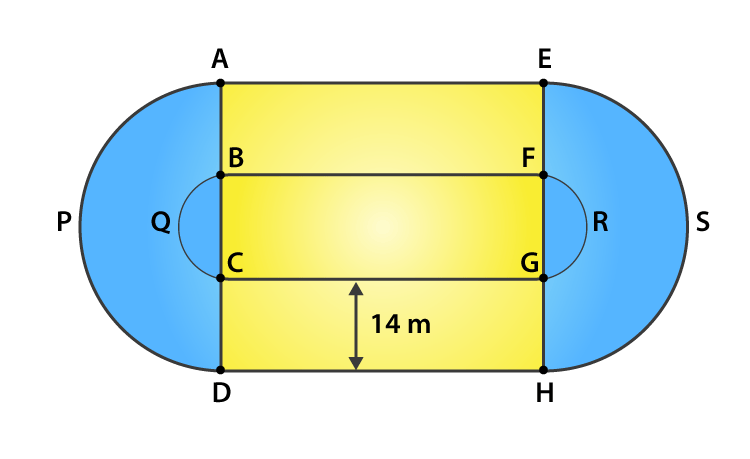Let the radius of the inner semi-circle = r

And that of the outer semi-circle = R

Given,

Length of the straight portion = 90 m

Width of the track = 14 m

The inner perimeter of the track = 400 m

But,

Inner perimeter of the track = BF + FRG + GC + CQB = 400

90 + πr + 90 + πr = 400

2 πr + 180 = 400

2 x 22/7 x r = 220

r = 35 m

So, the radius of the outer semi-circle = 35 + 14 = 49 m

Now,

Area of the track = 2[Area of the rectangle AEFB + Area of semi-circle APD – Area of semi-circle BQC]

= 2[90 x 14 + ½ x 22/7 x 492 – ½ x 22/7 x 352]

= 2[1260 + 11 x 7 x 49 – 11 x 5 x 35]

= 2 [1260 + 3773 – 1925]

= 3 x 3108

= 6216 m2

Thus,

The length of outer running track = AE + APD + DH + HSE

= 90 + πR + 90 + πR

= 180 + 2 πR

= 180 + 2 x 22/7 x 49

= 180 + 308

= 488 m

12. Find the area of Fig. 15.76 in square cm, correct to one place of decimal.

Solution: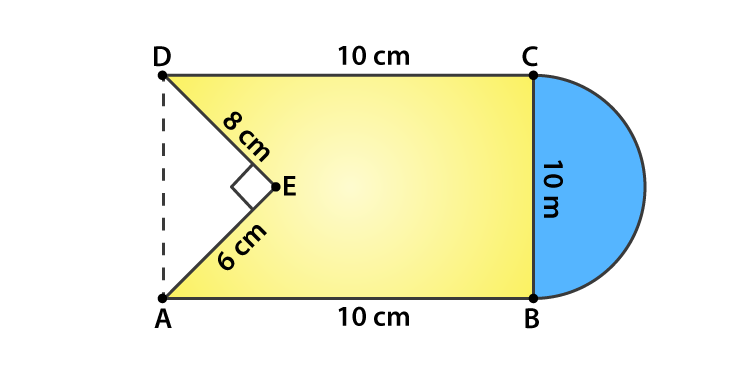The radius of the semi-circle = 10/2 = 5 cm

It’s seen that,

The area of figure = Area of square + Area of semi-circle – Area of triangle AED

= 10 x 10 + ½ πr2 – ½ x 6 x 8

= 100 + ½ (22/7)(5)2 – 24

= (700 + 275 – 168)/7

= (807)/7

= 115.3 cm2

13. From a rectangular region ABCD with AB = 20 cm, a right angle AED with AE = 9 cm and DE = 12 cm, is cut off. On the other end, taking BC as diameter, a semicircle is added on outside the region. Find the area of the shaded region. (π = 22/7)

Solution: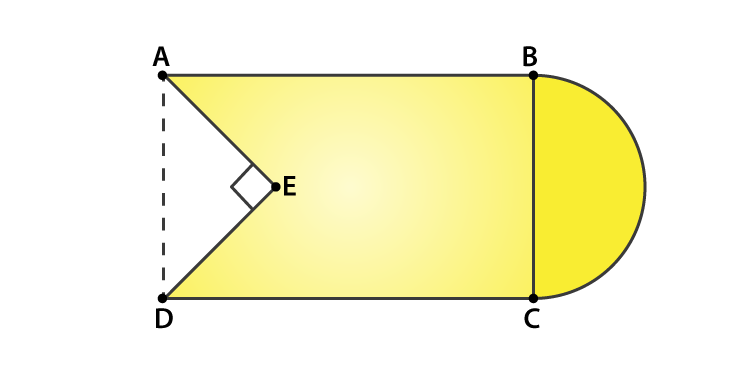Given,

Length of the rectangle ABCD = 20 cm

AE = 9 cm and DE = 12 cm

Now, using Pythagoras theorem in triangle AED

AD = √(AE2 + ED2) = √(92 + 122)

= √(81 + 144)

= √(225) = 15 cm

So, the area of the rectangle = 20 x 15 = 300 cm2

And, the area of the triangle AED = ½ x 12 x 9 = 54 cm2

The radius of the semi-circle = 15/2 = 7.5 cm

Area of semi-circle = ½ π(15/2)2 = ½ x 3.14 x 7.52 = 88.31 cm2

Thus,

The area of the shaded region = Area of the rectangle ABCD + Area of semi-circle – Area of triangle AED

= 300 + 88.31 – 54

= 334.31 cm2

14. From each of the two opposite corners of a square of side 8 cm, a quadrant of a circle of radius 1.4 cm is cut. Another circle of radius 4.2 cm is also cut from the center as shown in Fig. Find the area of the remaining (shaded) portion of the square. (Use π = 22/7)

Solution: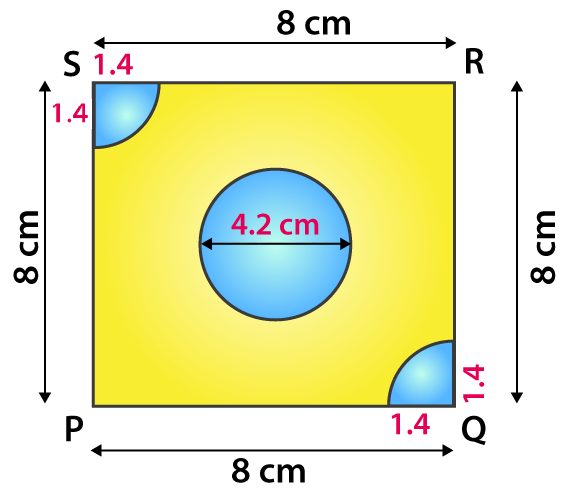Given,

Side of the square = 8 cm

Radius of circle = 4.2 cm

Thus,

Area of the shaded potion = Area of square – Area of circle – 2 x Area of the quadrant

= side2 – πr2 – 2 x ½ πr2

= 82 – π(4.2)2 – 2 x ½ π(1.4)2

= 64 – 22/7(4.2 x 4.2) – 22/7(1.4 x 1.4)

= 64 – 388.08/7 – 21.56/7

= 5.48 cm2

15. ABCD is a rectangle with AB = 14 cm and BC = 7 cm. Taking DC, BC and AD as diameters, three semi-circles are drawn as shown in the figure. Find the area of the shaded region.

Solution: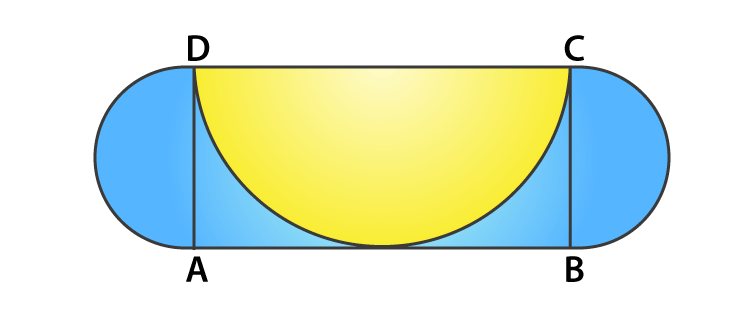Given,

ABCD is a rectangle with AB = 14 cm and BC = 7 cm

It’s seen that,

The area of shaded region = Area of rectangle ABCD + 2 x area of semi-circle with AD and BC as diameters – area of the semi-circle with DC as diameter

= 14 x 7 + 2 x ½ π(7/2)2 – ½ π(7)2

= 98 + 22/7 x (7/2)2 – ½ (22/7)(7)2

= 98 + 77/2 – 77

= 59.5 cm2

16. ABCD is rectangle, having AB = 20 cm and BC = 14 cm. Two sectors of 180° have been cut off. Calculate:

(i) the area of the shaded region.

(ii) the length of the boundary of the shaded region.

Solution: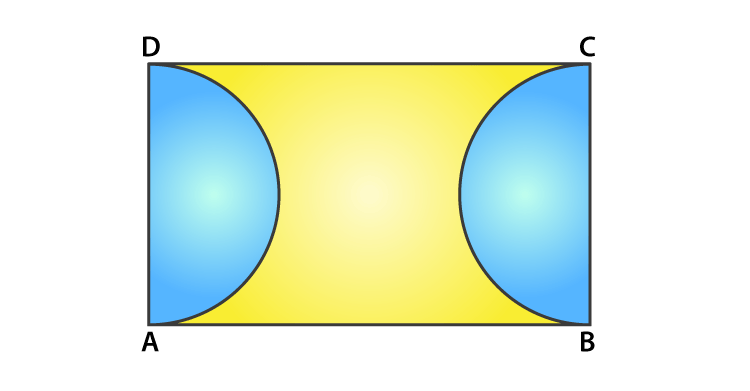Given,

Length of the rectangle = AB = 20 cm

Breadth of the rectangle = BC = 14 cm

(i) Area of the shaded region = Area of rectangle – 2 x Area of the semi-circle

= l x b – 2 x ½ πr2

= 20 x 14 – (22/7) x 72

= 280 – 154

= l26 cm2

(ii) Length of the boundary of the shaded region = 2 x AB + 2 x circumference of a semi-circle

= 2 x 20 + 2 x πr

= 40 + 2 x (22/7) x 7

= 40 + 44

= 84 cm

17. The square ABCD is divided into five equal parts, all having same area. The central part is circular and the lines AE, GC, BF and HD lie along the diagonals AC and BD of the square. If AB = 22 cm, find:

(i) the circumference of the central part.   (ii) the perimeter of the part ABEF.

Solution: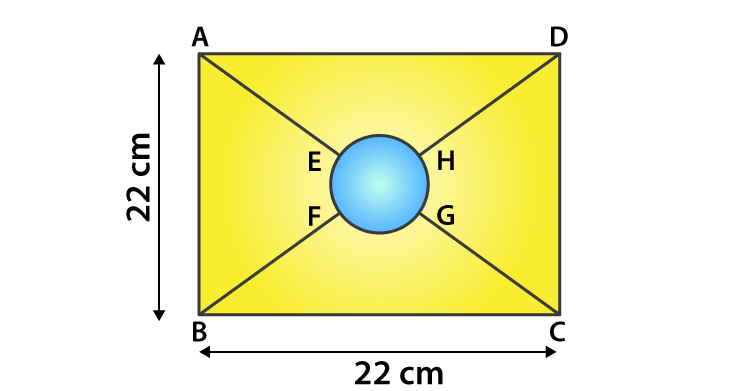Given,

Side of the square = 22 cm = AB

Let the radius of the centre part be r cm.

Then, area of the circle = 1/5 x area of the square

πr2 = 1/5 x 222

22/7 x r2 = (22 x 22)/ 5

r = 154/5 = 5.55 cm

(i) Circumference of central part = 2πr = 2(22/7)(5.55) = 34.88 cm

(ii) Let O be the center of the central part. Then, its clear that O is also the center of the square as well.

Now, in triangle ABC

By Pythagoras theorem

AC2 = AB2 + BC2 = 222 + 222 = 2 x 222

AC = 22√2

Since diagonals of a square bisect each other

AO = ½ AC = ½ (22√2) = 11√2 cm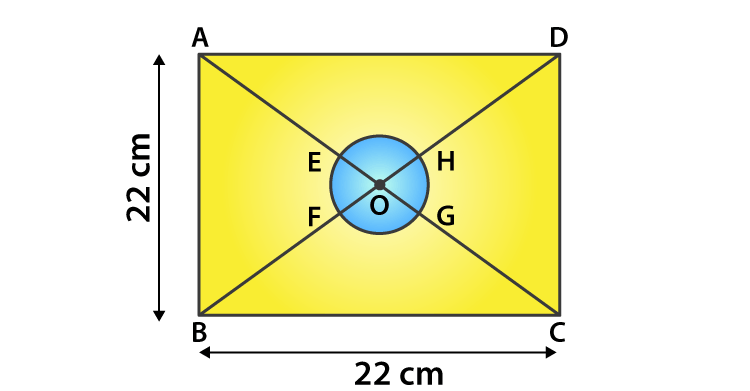And,

AE = BF = OA – OE = 11√2 – 5.55 = 15.51 – 5.55 = 9.96 cm

EF = ¼(Circumference of the circle) = 2πr/4

= ½ x 22/7 x 5.55 = 8.72 cm

Thus, the perimeter of the part ABEF = AB + AE + EF + BF

= 22 + 2 x 9.96 + 8.72

= 50.64 cm

18. In figure, find the area of the shaded region. (Use π = 3.14)

Solution: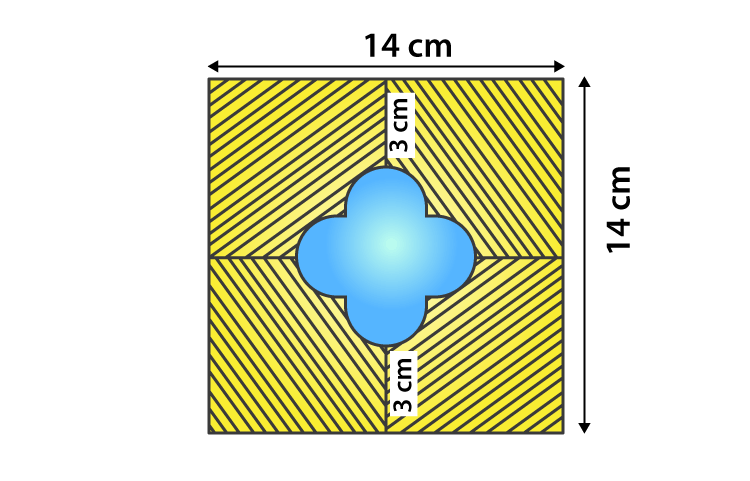The side of the square = 14 cm

So, it’s area = 142 = 196 cm2

Let’s assume the radius of each semi-circle be r cm.

Then,

r + 2r + r = 14 – 3 – 3

4r = 8

r = 2

The radius of each semi-circle is 2 cm.

Area of 4 semi-circles = (4 x ½ x 3.14 x 2 x 2) = 25.12 cm2

Now, length of side of the smaller square = 2r = 2 x 2 = 4 cm

Thus, the area of smaller square = 4×4 = 16 cm2

Area of unshaded region = Area of 4 semi-circles + Area of smaller square

= (25.12 + 16) = 41.12 cm2

Therefore,

The area of shaded region = Area of square ABCD – Area of unshaded region

= (196 – 41.12) = 154.88 cm2

19. OACB is a quadrant of a circle with center O and radius 3.5 cm. If OD = 2 cm, find the area of the (i) quadrant OACB (ii) shaded region.

Solution: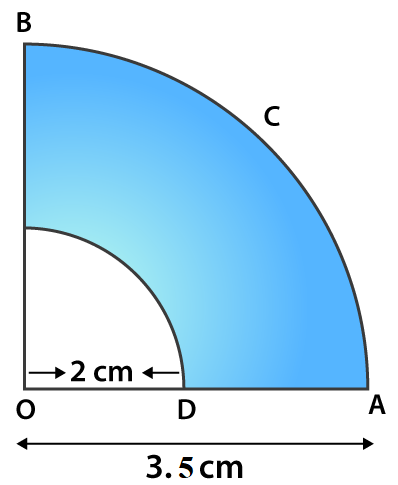Given,

(i) Area of quadrant OACB = ¼ πR2

= ¼ (22/7)(3.5)2

= 269.5/28 = 9.625 cm2

= ¼ π(R2 – r2)

= ¼ (22/7)(3.52 – 22)

= ¼ (22/7)(12.25 – 4)

= ¼ (22/7)(8.25)

= 6.482 cm2

20. A square OABC is inscribed in a quadrant OPBQ of a circle. If OA = 21 cm, find the area of the shaded region.

Solution:Given,

Side of the square = 21 cm = OA

Area of the square = OA2 = 212 = 441 cm2

Diagonal of the square OB = √2 OA = 21√2 cm

And, from the fig. its seen that

The diagonal of the square is equal to the radius of the circle, r = 21√2 cm

So, the area of the quadrant = ¼ πr2 = ¼ (22/7)(21√2)2 = 693 cm2

Thus,

The area of the shaded region = Area of the quadrant – Area of the square

= 693 – 441

= 252 cm2

21. OABC is a square of side 7 cm. If OAPC is a quadrant of a circle with centre O, then find the area of the shaded region.

Solution: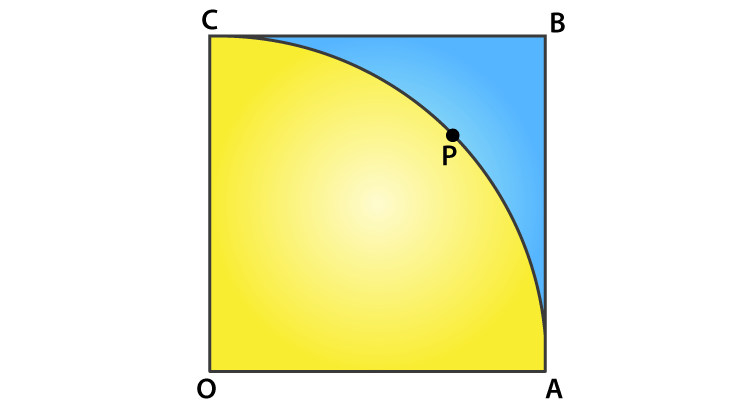Given,

OABC is a square of side 7 cm

So, OA = AB = BC = OC = 7 cm

Area of square OABC = side2 = 72 = 49 cm2

And given, OAPC is a quadrant of a circle with centre O.

So, the radius of the quadrant = OA = OC = 7 cm

Area of the quadrant OAPC = 90/360 x πr2

= ¼ x (22/7) x 72

= 77/2 = 38.5 cm2

Thus,

Area of shaded portion = Area of square OABC – Area of quadrant OAPC

= (49 – 38.5) = 10.5 cm2

22. OE = 20 cm. In sector OSFT, square OEFG is inscribed. Find the area of the shaded region.

Solution: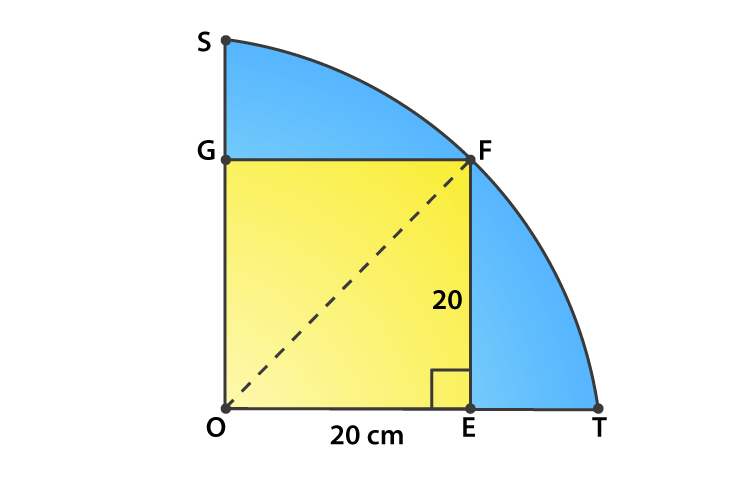It’s seen that, OEFG is a square of side 20 cm.

So its diagonal = √2 side = 20√2 cm

So,

Area of the shaded portion = Area of quadrant – Area of square

= ¼ πr2 – side2

= ¼ (22/7)(20√2)2 – (20)2

= ¼ (22/7)(800) – 400

= 400 x 4/7 = 1600/7 = 228.5 cm2

23. Find the area of the shaded region in Fig., if AC = 24 cm, BC = 10 cm and O is the center of the circle

Solution: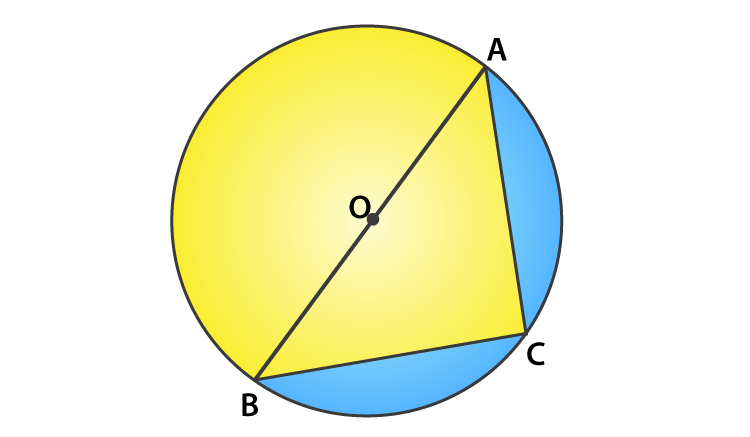Given,

AC = 24 cm and BC = 10 cm

Since, AB is the diameter of the circle

Angle ACB = 90o

So, using Pythagoras theorem

AB2 = AC2 + BC2 = 242 + 102 = 576 + 100 = 676

AB = √676 = 26 cm

Thus, the radius of the circle = 26/2 = 13 cm

The area of shaded region = Area of semi-circle – Area of triangle ACB

= ½ πr2 – ½ x b x h

= ½ (22/7)132 – ½ x 10 x 24

= 265.33 – 120

= 145.33 cm2

24. A circle is inscribed in an equilateral triangle ABC of side 12 cm, touching its sides (fig.,). Find the radius of the inscribed circle and the area of the shaded part.

Solution: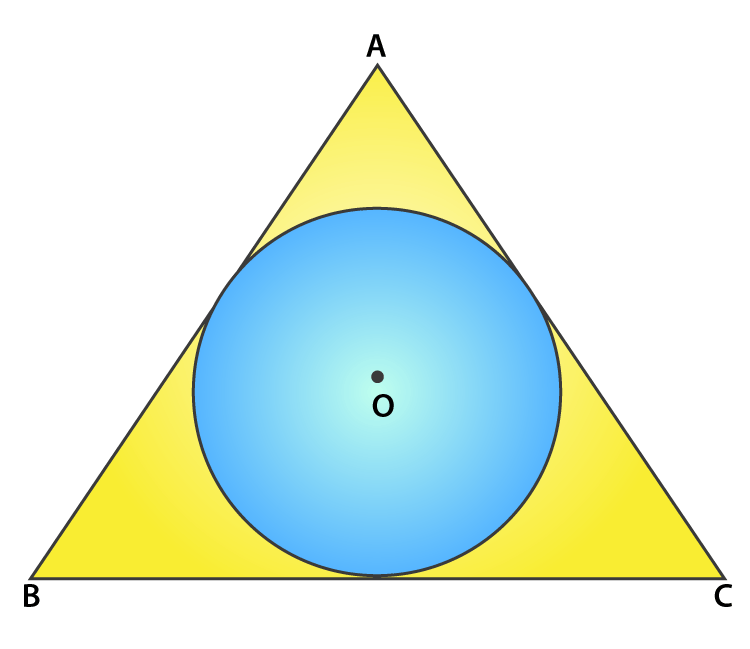Given,

An equilateral triangle of side = 12 cm

Area of the equilateral triangle = √3/4(side)2

= √3/4(12)2 = 36√3 cm2

Perimeter of triangle ABC = 3 x 12 = 36 cm

So,

The radius of incircle = Area of triangle/ ½ (perimeter of triangle)

= 36√3/ ½ x 36

= 2√3 cm

Therefore,

Area of the shaded part = Area of equilateral triangle – Area of circle

= 36√3 – πr2

= 36(1.732) – (3.14)(2√3)2

= 62.352 – 37.68

= 24.672 cm2

25. In fig., an equilateral triangle ABC of side 6 cm has been inscribed in a circle. Find the area of the shaded region. (Take π = 3.14).

Solution: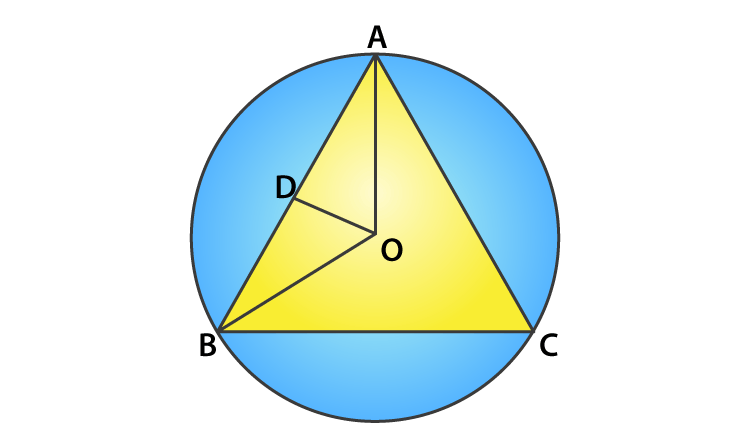Given,

Side of the equilateral triangle = 6 cm

And,

The area of the equilateral triangle = √3/4(side)2

= √3/4(6)2

= √3/4(36)

= 9√3 cm2

Let us mark the center of the circle as O, OA and OB are the radii of the circle.

In triangle BOD,

sin 60o = BD/ OB

√3/2 = 3/ OB

OB = 2√3 cm = r

Therefore,

The area of shaded region = Area of the circle – area of the equilateral triangle

= πr2 – 9√3

= 3.14 x (2√3)2 – 9√3

= 3.14 x 12 – 9 x 1.732

= 37.68 – 15.588

= 22.092 cm2